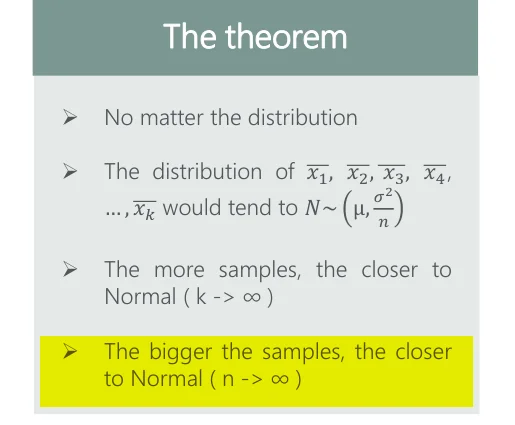01 Aug 2023

Posted on:

07 Apr 2023

0

# In CLT theoram: The bigger the samples, the closer to Normal ( n -> ∞ )

In the Pdf "Statistics - Course Notes. Page number: 21 titled "Central Limit Theorem"

The last point of the box titled "The theorem" says: The bigger the samples, the closer
to Normal ( n -> ∞ )

Got, it as with the bigger sample we would have more possibility of being accurate which means closer to the value of normal. But the notational representation in bracket  ( n -> ∞ ) says that the sample size should tend to infinity. But I think it is incorrect as the population size is denoted as capital N. This means sample size lowercase n -> N for getting correct. How can any sample become greater than the size of the population itself?

Is my analogy correct?Instructor
Posted on:

10 Apr 2023

0

Hello,

This is correct. If the sample size n tends to infinity then the distribution of the sample will approximate the Normal distribution.

Best,

Ned

Posted on:

01 Aug 2023

0

I understood it as (n -> ∞) means as many samples as possible. assuming you have taken  ∞ samples, then you could have taken everything in the population. so n -> ∞ is just same as n -> N